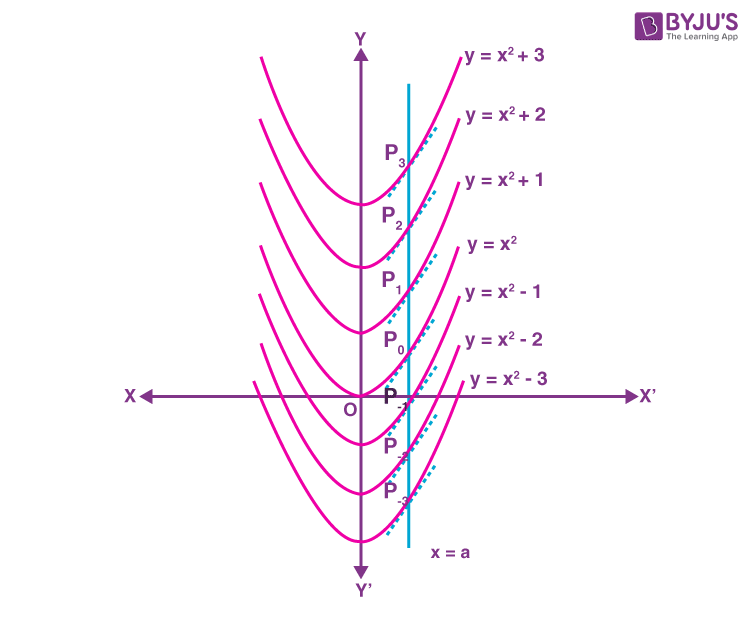# Geometrical Interpretation of Indefinite Integral

In Calculus, integration is the process of finding the antiderivatives of a function. In other words, we can say that integration is the inverse process of differentiation. While integrating the function, we will get the constant of integration known as arbitrary constant C. For different values of C, we will get different integrals. But those integrals are geometrically similar. Here, we are going to discuss the geometrical interpretation of indefinite integral in detail with an example.

## Geometrical Interpretation of Indefinite Integral Example

Assume the function, f(x) = 2x. Now, integrate the function, we get

∫ f(x) dx = ∫ 2x dx

∫ 2x dx = x2 + C

Where C is called the arbitrary function, which represents the family of integrals.

We know that for different values of C, we get different integral values. But geometrically, those integral values are similar. It means that, by assigning different values to the arbitrary constant, we will get the different members of the family, which constitute the indefinite integral. Thus, each integral represents the parabola with its axis along the y-axis.

For example,

If C = 0, then we get y = x2, which is a parabola with its vertex on the origin.

If C = 1, then we get y= x2+1, which can be obtained by shifting the parabola one unit along the y-axis in the positive direction.

If C = 2, then we have = y = x2+2, and it can be obtained by shifting the parabola to two units in the positive y-axis.

Similarly, if for C = -1, we get y = x2 – 1, which can be obtained by shifting the parabola one unit along the y-axis in the negative direction.

Hence, for each positive value of C, each parabola of the family has a vertex along the y-axis in a positive direction. And for each negative value of C, each parabola of the family has a vertex along the negative y-axis direction.

The following graph represents the geometrical interpretation of the indefinite integral ∫ 2x dx = x2 + C.Here, the line x=a intersects all the parabolas. It is observed that a>0, and the same is true when a is less than 0. The line x=a intersect the parábola, y=x2, y= x2+1, y= x2+2, y= x2+3, y=x2-1, y=x2-2, y=x3-3 at the point P, P1, P2, P3, P-1, P-2, P-3 respectively. Hence, dy/dx at these points are equal to 2a. This scenario indicates that the tangent to these parabolic curves at these points are parallel.

Hence, the equation ∫ 2x dx = x2 + C =y defines that the different values of arbitrary constants C will correspond to different members of the family, which can be obtained by shifting one of the curves parallel to itself. This is known as the geometrical interpretation of indefinite integral.